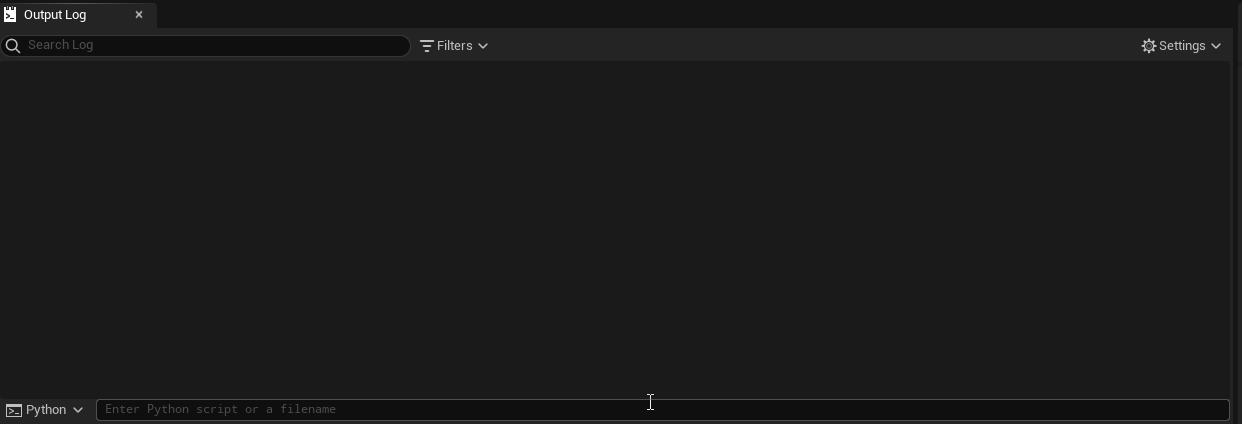Beside this tutorial How to manipulate User Defined ENum, Struct, DataTable with Python in Unreal Engine，there is also a common requirement to use Python to handle materials and material functions in Unreal Engine.

• Create/Delete Material and Material Expression
• Connect The Expressions
• Get deep detail from expressions and material, for instance, shadermap and hlsl code.

The MaterialEditingLibrary in Unreal Engine 5 has dozens of material APIs for python, and can do lots with material and MF, but there also something that can't handle well.

For example:

• Can't connect expressions to the material property: "World Position offset" The Enum value MP_WorldPositionOffset was marked as "Hidden" in c++, so we can use this Enum in python.

• Can ddd Input/Output pins for Material Expressions: Get/SetMaterialAttributes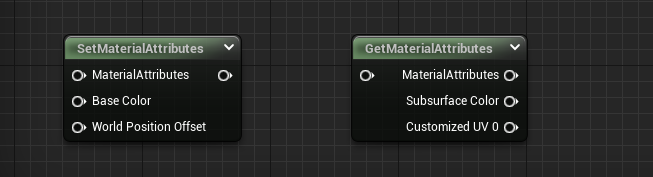I can understand UE has lots of good reasons for hiding that Enums, which can avoid a lot of troubles. For instance, if we foolishly set the shader mode to MSM_Strata (DisplayName="Strata", Hidden), the editor will crash immediately. But hiding the options and enumerations that users can manipulate through the UI also adds an extra workload for automation task. And that's what TAPython is for.

Right now，with MaterialEditingLibrary and PythonMaterialLib of TAPython，we can script almost every material operations in Python. My goal is to make it 100% scriptable and automatable.

## Create¶

### Create a Material¶

The code below will create a material named M_CreatedByPython at "/Content/CreatedByPython".

``````asset_tools = unreal.AssetToolsHelpers.get_asset_tools()
my_mat = asset_tools.create_asset("M_CreatedByPython", "/Game/CreatedByPython", unreal.Material, unreal.MaterialFactoryNew())
unreal.EditorAssetLibrary.save_asset(my_mat.get_path_name())
``````

### Create a Material Function¶

Creating a material function is similar to creating a material.

``````asset_tools = unreal.AssetToolsHelpers.get_asset_tools()
my_mf = asset_tools.create_asset("MF_CreatedByPython", "/Game/CreatedByPython", unreal.MaterialFunction, unreal.MaterialFunctionFactoryNew())
``````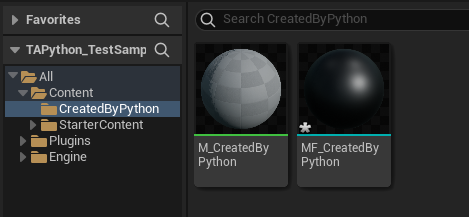• Add a simple material expression node

Note that most of the methods or functions described in this article will not trigger a refresh of the material editor UI. Therefore, if you open the current material in the Material editor, you need to close the window and then open it again to see the newly added node.

``````node_add = unreal.MaterialEditingLibrary.create_material_expression(my_mat, unreal.MaterialExpressionAdd, node_pos_x=-200, node_pos_y=0)
``````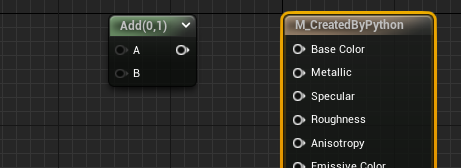Adding a material node to a material function is similar, except that the method called is unreal.MaterialEditingLibrary.create_material_expression_in_function.

``````node_add = unreal.MaterialEditingLibrary.create_material_expression_in_function(my_mf, unreal.MaterialExpressionAdd)
``````

Adding a TextureSample Expression，and set the texture using set_editor_property

``````node_tex = unreal.MaterialEditingLibrary.create_material_expression(my_mat, unreal.MaterialExpressionTextureSampleParameter2D
, node_pos_x=-600, node_pos_y=0)

node_tex.set_editor_property("texture", texture_asset)
``````• Add a Material Function node

The class type of Material Function Expression is：MaterialExpressionMaterialFunctionCall，Then we also need to set the MF asset, via set_editor_property.

``````node_break = unreal.MaterialEditingLibrary.create_material_expression(my_mat, unreal.MaterialExpressionMaterialFunctionCall, -600, 300)
``````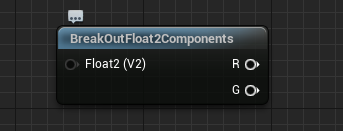The above examples using the MaterialEditingLibrary that comes with the Unreal engine. Some of the following will require PythonMaterialLib in TAPython.

Some material nodes are special that they have input and output pins that user can add. The data of the input and output pins are stored in FGuid. Simply setting the value of that will not trigger the adding pins task and will cause an error.

So, we need to use the add_input_at_expression_set_material_attributes in PythonMaterialLib which will both add the input and pins for material editor.

``````node_sma = unreal.MaterialEditingLibrary.create_material_expression(my_mat, unreal.MaterialExpressionSetMaterialAttributes, node_pos_x=500, node_pos_y=0)
property_names = ["MP_Specular", "MP_Normal", "MP_WorldPositionOffset", "MP_CustomData0", "MP_CustomizedUVs0"]

for mp_name in property_names:

# same with MaterialExpressionGetMaterialAttributes
node_gma = unreal.MaterialEditingLibrary.create_material_expression(my_mat, unreal.MaterialExpressionGetMaterialAttributes, node_pos_x=200, node_pos_y=0)
for mp_name in property_names:
``````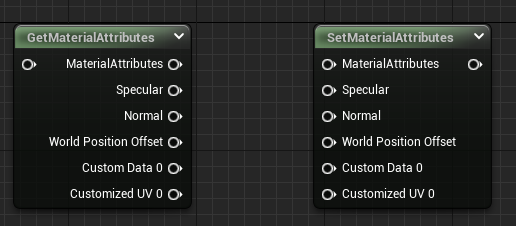## Connect¶

### Connect Material Expression to Material Property¶

• Connect a material expression's output to Material Property
``````# use MaterialEditingLibrary
, from_output_name=""
, property_=unreal.MaterialProperty.MP_BASE_COLOR)
``````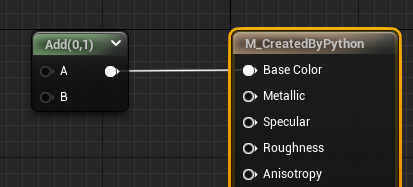• Connect expression's output to some "Hidden" Material Property, for instance, WorldPositionOffset

As of now(UE 5.0.3), unreal.MaterialProperty does not contain any enums items such as MP_WorldPositionOffset that are marked hidden in CPP, so we can't connect expression to it with the function above.

Then we can use the function of the same name that in PythonMaterialLib. The third argument of this function's type changed to string.

``````# use PythonMaterialLib
, from_output_name=""
, material_property_str="MP_WorldPositionOffset")
``````

tips: When the input or output node names from_output_name or to_input_name are empty strings, the first input or output pin is used by default.If a node has multiple outputs, you can use this method to see which OUTPUT_names a specific node has：GetMaterialExpressionOutputNames

• Connect between Material Expressions
``````unreal.MaterialEditingLibrary.connect_material_expressions(from_expression=node_tex, from_output_name="", to_expression=node_add, to_input_name="A")
``````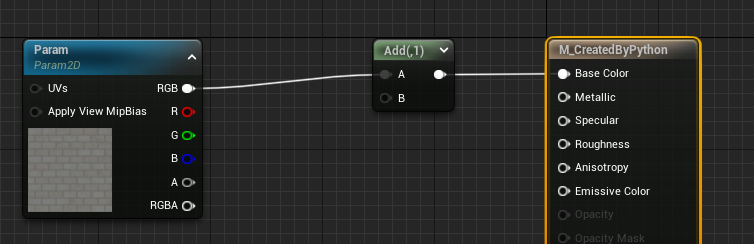## Delete¶

### Delete Material Expression¶

``````unreal.PythonMaterialLib.delete_material_expression(my_mat, node_need_be_delete)
``````

### Disconnections¶

• Disconnect with Material Property
``````unreal.PythonMaterialLib.disconnect_material_property(my_mat, material_property_str="MP_BaseColor")
``````
• Disconnect between Material Expressions

The output pin of a material expression can connect to multiple other expressions, but the input pin of it is unique. So when we want to disconnect the connections between expressions, only to specify the expression which the connection connected to and its input name.

``````unreal.PythonMaterialLib.disconnect_expression(node_add, "A")
``````

## Layout the Expressions¶

In addition to specifying the location of the material node when it is created, we can also apply automatic layout to the material after it has been connected.

• Auto layout
``````unreal.MaterialEditingLibrary.layout_material_expressions(my_mat)
``````
• Specify the node location when creating the node
``````unreal.MaterialEditingLibrary=create_material_expression( material, expression_class, node_pos_x=x, node_pos_y=y):
``````

## Query¶

### Get the instance of Material Expression (node in material)¶

We can add context menus in Material Editor with TAPython 1.0.8, and get the selections of the expressions. More descriptions can be found here. It's very useful for the python scripts that handle the material graphs.

``````    "OnMaterialEditorMenu": {
"name": "Python Menu On Material Editor",
"items":
[
{
"name": "TA Python Material Example",
"items": [
{
"name": "Print Editing Material / MF",
"command": "print(%asset_paths)"
},
{
"name": "Log Editing Nodes",
},
{
"name": "Selected Nodes --> global variable _r",
},
{
"name": "Selected Node --> _r",
}
]
}
]
},
``````

Below example will log the selected expression's brief info with the menu item "Log Editing Nodes"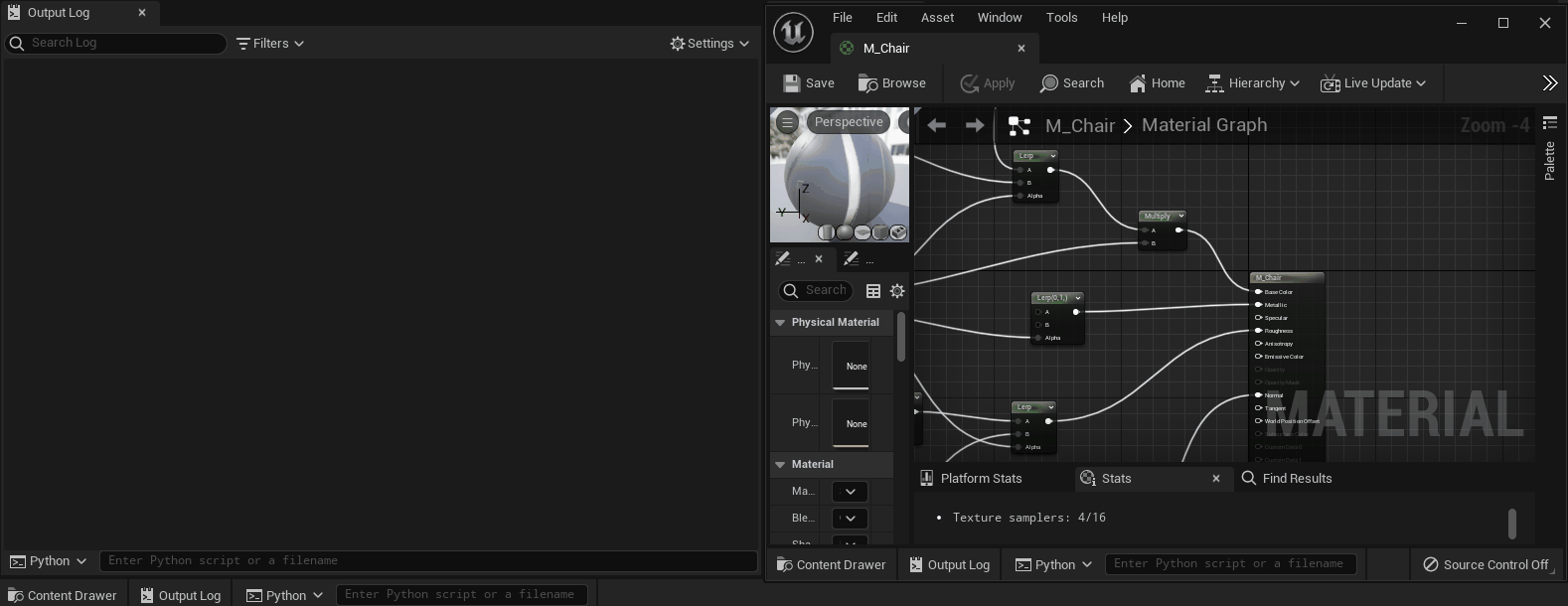Menu item "Selected Node --> global variable _r" will get the selected expression and assign them to the global variable "_r". And we can print out its property or show all the details of it with ObjectDetailViewer.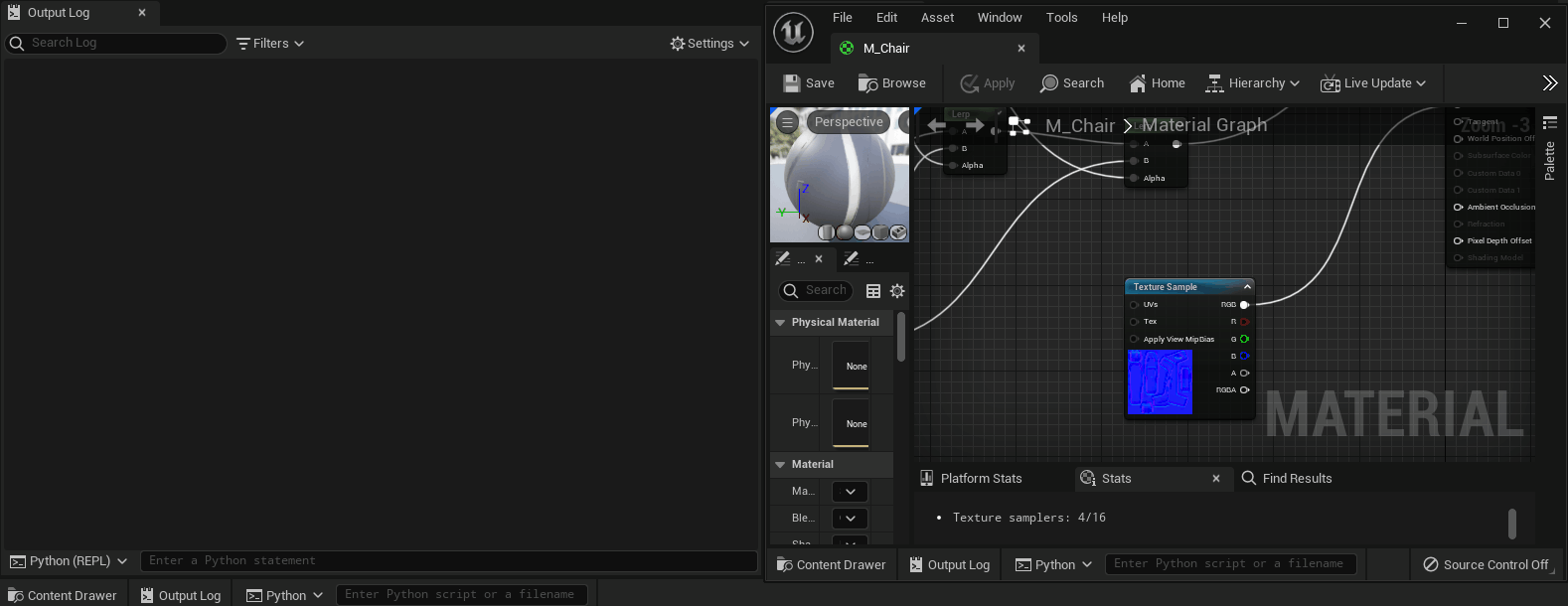### Get properties of the Material Expression¶

• Get the input names

Get the input pin's names of the material expression

``````unreal.PythonMaterialLib.get_material_expression_input_names(some_node)
``````
• Get the output names
``````unreal.PythonMaterialLib.get_material_expression_output_names(some_node)
``````
• Get the captions
``````unreal.PythonMaterialLib.get_material_expression_captions(some_node)
``````
• print out a brief of the material expression
``````unreal.PythonMaterialLib.log_material_expression(some_node)
``````The type of "Input Type" and "Output Type" in above image is EMaterialValueType, which can be found in Utilities.Utils. Value 15 means MCT_Float1|MCT_Float2|MCT_Float3|MCT_Float4.

``````class EMaterialValueType(IntFlag):
MCT_Float1          = 1,
MCT_Float2          = 2,
MCT_Float3          = 4,
MCT_Float4          = 8,
MCT_Texture2D       = 1 << 4,
....
MCT_VoidStatement   = 1 << 22
``````
• Print out node connections in the material in a tree form

The number in square brackets are the indexes of the material expressions. The expressions can be get via get_material_expressions.

``````unreal.PythonMaterialLib.log_mat(my_mat)
``````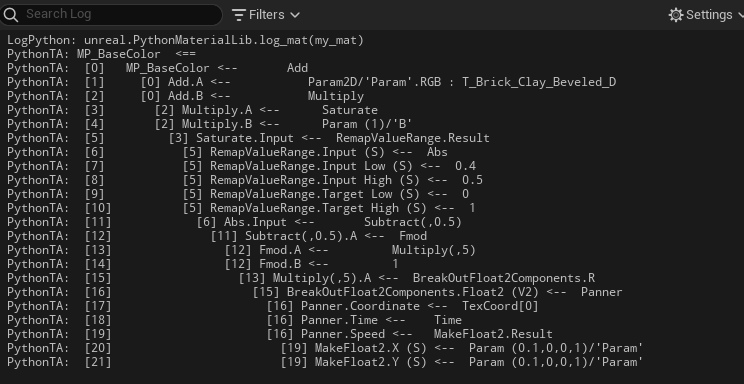The material function also has a similar function

``````unreal.PythonMaterialLib.log_mf(my_mf)
``````

### Get the material expressions and the connections¶

• Get all expressions of the material
``````all_expressions = unreal.PythonMaterialLib.get_material_expressions(my_mat)
``````
• Get all expressions of the material function
``````all_expressions_in_mf = unreal.PythonMaterialLib.get_material_function_expressions(my_mf)
``````
• Get the connections between expressions in the material

Below code will return all the connections in a list, the type of item is TAPythonMaterialConnection.

``````for connection in unreal.PythonMaterialLib.get_material_connections(my_mat)
print(connection)
``````
TAPythonMaterialConnection(StructBase):
``````
class TAPythonMaterialConnection(StructBase):
r"""
TAPython Material Connection

**C++ Source:**

- **Plugin**: TAPython
- **Module**: TAPython
- **File**: PythonMaterialLib.h

**Editor Properties:** (see get_editor_property/set_editor_property)

- ``left_expression_index`` (int32):  [Read-Write] Left Expression Index:
The index of material expression in source material's expressions, which the connection from
- ``left_output_index`` (int32):  [Read-Write] Left Output Index:
The index of output in the expression
- ``left_output_name`` (str):  [Read-Write] Left Output Name:
The name of output pin
- ``right_expression_index`` (int32):  [Read-Write] Right Expression Index:
The index of material expression in source material's expressions, which the connection to
- ``right_expression_input_index`` (int32):  [Read-Write] Right Expression Input Index:
The index of input in the expression
- ``right_expression_input_name`` (str):  [Read-Write] Right Expression Input Name:
The name of input pin
``````

Properties of the connection：

• left_expression_index
• left_output_index
• left_output_name
• right_expression_index
• right_expression_input_index
• right_expression_input_name

"Left_expression_index" and "right_expression_index" are the node's index of the left and right expressions beside the "connection wire".

## Export¶

### Export Material in JSON format¶

Below codes will export the material including its expressions and connections between them into a json file.

This function is essentially the same as exporting materials as T3D or COPY, but in the more general JSON format. In this way, we can get every detail about the nodes of material. Then we can analyze, optimize, and do other cool things.

For instance, exporting UE5's material to UE4, or other internal engine which modifies the serialize of the assets.

``````    # export the material content to a JSON file
content_in_json = unreal.PythonMaterialLib.get_material_content(my_mat)
with open("file_path_of_json_file", 'w') as f:
f.write(content_in_json)
``````

And there will be an example in this repo showing how to re-create the material from exported Json file.

### Get HLSL code¶

We can get the material's HLSL code under FeatureLevel: SM5.

``````print(unreal.PythonMaterialLib.get_hlsl_code(my_mat))
``````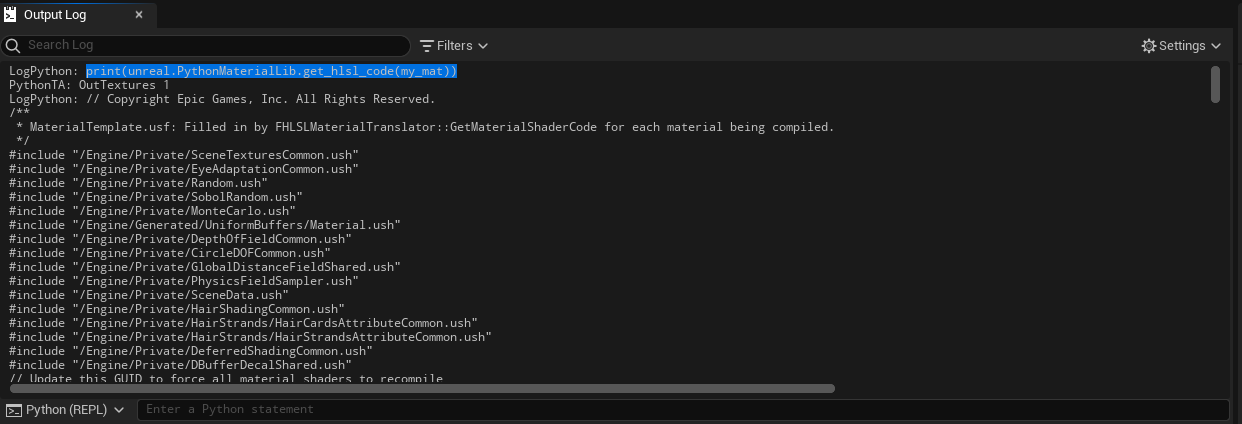### Get shadermap in JSON format¶

Below data of the material's shadermap will be exported in JSON format, for future analysis.

• Name
• FunctionName
• VertexFactoryType
• FrequencyType
• PermutationId
``````unreal.PythonMaterialLib.get_shader_map_info(my_mat, "PCD3D_ES3_1")